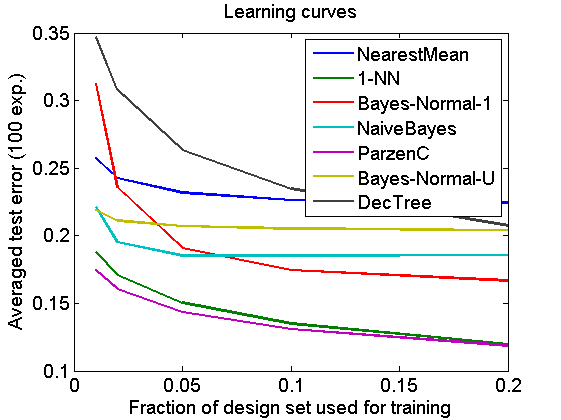# crossval_ex

Experiment to compare some crossvalidation procedures on the basis of their ability to rank the expected perfomance of some classifiers for various sizes of the training set. The experiment is based on the satelliite dataset.

The following crossvalidation procedures are compared:

• kohavi10 - single 10-fold crossvalidation, see 
• kohavi10x10 - 10 times 10-fold crossvalidation, see 
• dietterich5x2 - 5 times 2-fold crossvalidation, see ,
• dps8 - single 8-fold density preserving split, see 

References:

1. R. Kohavi, A Study of Cross-Validation and Bootstrap for Accuracy Estimation and Model Selection IJCAI, pp. 1137-1145, Morgan Kaufmann, 1995. download
2. T.G. Dietterich, Approximate Statistical Tests for Comparing Supervised Classification Learning Algorithms, Neural Computation, 10(7), pp. 1895-1924, 1998. download
3. E. Alpaydin, Combined 5 x 2 cv F Test for Comparing Supervised Classification Learning Algorithms, Neural Computation, 11(8), pp. 1885-1892, 1999 download
4. M. Budka, B. Gabrys, Correntropy-based density-preserving data sampling as an alternative to standard cross-validation, IJCNN2010, 1-8 download

PRTools and PRDataSets should be in the path

## Initialise

```a       = satellite;
a       = setprior(a,getprior(a,0));  % give classes equal priors
alfs    = [0.01 0.02 0.05 0.1 0.2];   % part of design set used for training
clsfs   = {nmc,knnc([],1),ldc,naivebc,parzenc,udc,treec}; % classifiers
procs   = {'kohavi10','kohavi10x10','dietterich5x2','dps8'};
repeats = 100;                        % number of generated training sets
nprocs  = numel(procs);               % number of crossval prpcedures
nclsfs  = numel(clsfs);               % number of classifiers
nalfs   = numel(alfs);                % number of training set sizes
eclsfs  = zeros(repeats,nalfs,nclsfs);% true classifier performances
R       = zeros(repeats,nprocs,nalfs);% final Borda count differences
```
```
*** You are using one of the datasets distibuted by PRTools. Most of them
*** are publicly available on the Internet and converted to the PRTools
*** format. See the Contents of the datasets directory (HELP PRDATASETS)
*** or the load routine of the specific dataset to retrieve its source.

```

## Run all crossvalidations

• various training set sizes (e.g. 5)
• various train / test splits (e.g. 100)
• various crossvalidation procedures (4)
• all classifiers (e.g. 7) This may take several hours
```q = sprintf('Running %i crossvals: ',repeats*nprocs*nalfs);
[NUM,STR,COUNT] = prwaitbarinit(q,repeats*nprocs*nalfs);
for n = 1:nalfs                     % run over training set sizes
alf = alfs(n);
for r=1:repeats                   % repeat repeats times
randreset(r);                   % take care of reproducability
[s,t] = gendat(a,alf);          % full training set s, large test set t
eclsfs(r,n,:) = cell2mat(testc(t,s*clsfs)); % train, test all classifiers
[dummy,T] = sort(squeeze(eclsfs(r,n,:))');
T(T) = [1:nclsfs];              % true Borda count classifiers
for j=1:nprocs                  % run over crossval procedures
COUNT = prwaitbarnext(NUM,STR,COUNT);
proc = procs{j};
switch lower(proc)
case 'kohavi10'             % single 10-fold crossval, all clsfs
exval = prcrossval(s,clsfs,10,1);
case 'kohavi10x10'          % 10 times 10-fold crossval, all clsfs
exval = prcrossval(s,clsfs,10,10);
case 'dietterich5x2'        % 5 times 2-fold crossval, all clsfs
exval = prcrossval(s,clsfs,2,5);
case 'dps8'                 % single 8-fold density preserving split
exval = prcrossval(s,clsfs,8,'dps');
otherwise
error('Unknown crossvalidation procedure')
end
[dummy,S] = sort(exval);
S(S) = [1:nclsfs];            % estimated Borda counts
R(r,j,n) = sum(abs(T-S))/2;   % Borda count differences
end
end
end
```

## Learning curves

```figure;
plot(alfs,squeeze(mean(eclsfs)));
legend(getname(clsfs));
xlabel('Fraction of design set used for training')
ylabel(['Averaged test error (' num2str(repeats) ' exp.)'])
title('Learning curves')
fontsize(14)
linewidth(1.5)
```## Table with results

```fprintf('    procedure  |');
fprintf('    %4.2f     |',alfs);
fprintf('\n  ');
fprintf('%s',repmat('-------------|',1,nalfs+1));
fprintf('\n');
for j=1:nprocs
fprintf(' %13s |',procs{j});
for n=1:nalfs
fprintf(' %4.2f (%4.2f) |',mean(R(:,j,n)),std(R(:,j,n))/sqrt(repeats));
end
fprintf('\n');
end
```
```    procedure  |    0.01     |    0.02     |    0.05     |    0.10     |    0.20     |
-------------|-------------|-------------|-------------|-------------|-------------|
kohavi10 | 3.18 (0.14) | 2.38 (0.12) | 1.43 (0.10) | 1.01 (0.09) | 1.14 (0.08) |
kohavi10x10 | 2.73 (0.12) | 2.05 (0.13) | 1.25 (0.09) | 0.97 (0.08) | 1.04 (0.08) |
dietterich5x2 | 2.43 (0.11) | 2.25 (0.11) | 1.61 (0.10) | 1.36 (0.10) | 1.67 (0.10) |
dps8 | 2.92 (0.13) | 2.26 (0.13) | 1.37 (0.09) | 0.98 (0.08) | 1.17 (0.08) |
```

This table shows how well the four crossvalidation procedures are able to predict the ranking of the performances of 7 classifiers trained by the total training set. The columns refer to different sizes of the training set. They are fractions of the total design set of 6435 objects.

The numbers in the table are the averaged sums of the Borda count differences between the estimated ranking (by the corresponding procdure) and the true ranking, divided by two. A value of 0 stands for equal rankings and 12 for fully opposite rankings. A single swap between two classifiers results in a value of 1. In brackets the standard deviations in the estimated avarages.

The 10 x 10-fold crossvalidation needs about 10 times more computing time than the three other procedures. Except for the smallest training sizes, it performs always better than the other three. The dietterich5x2 procedure starts as the best one but is for larger training sets the worst. Here it suffers from the fact that in the crossvalidation it uses training sets that have just half the size of the final set for which it has to predict the classifier performances. Some learning curves are not yet saturated. This explains also why the final performances based on using 20% of the design set are worse then at 10%: At 20% there is more confusion between the classifier rankings.